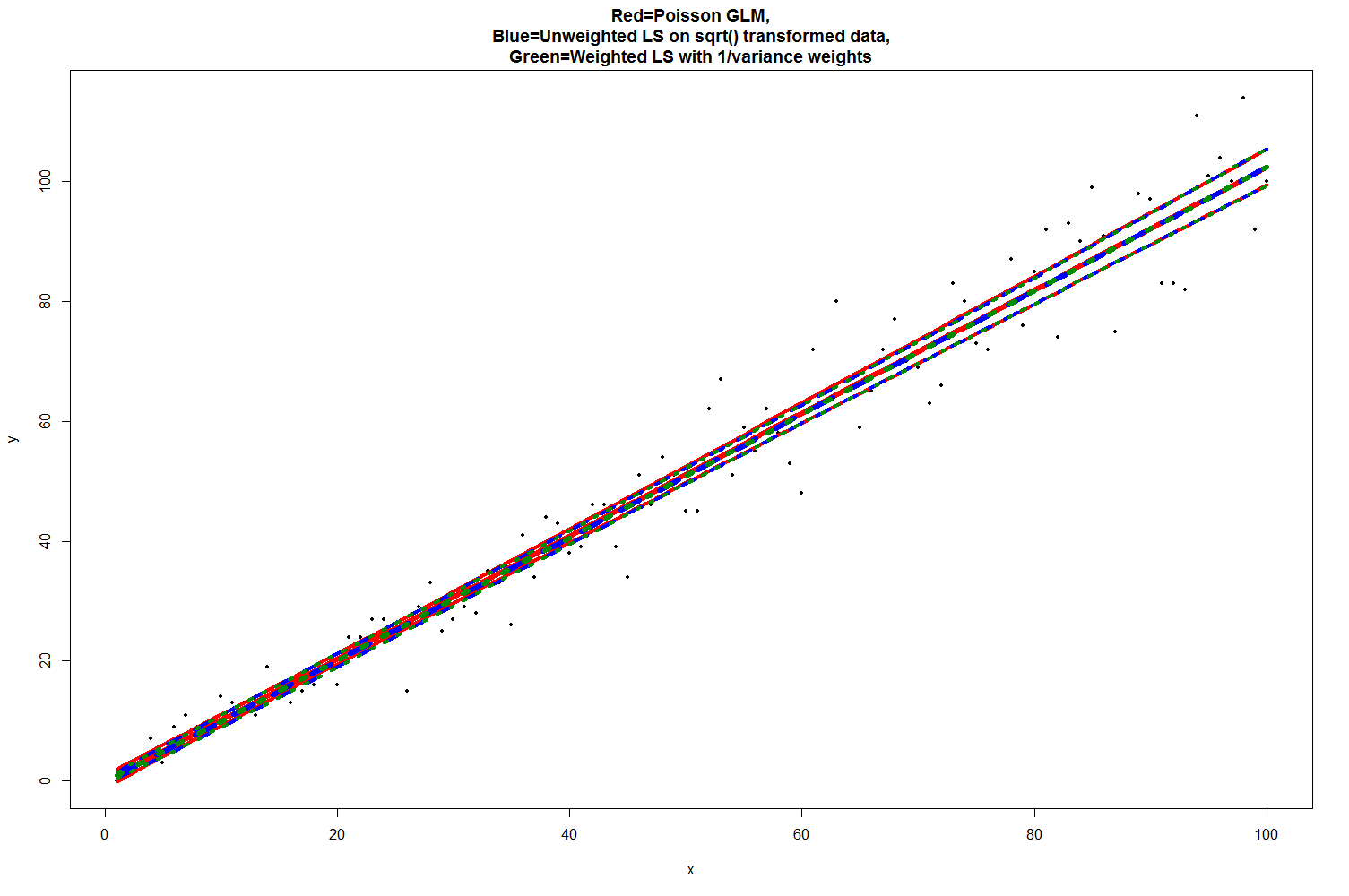# #StackBounty: #r #heteroscedasticity #poisson-regression #weighted-regression Closest approximation of a Poisson GLM using weighted lea…

### Bounty: 100

I have an application where I would like to approximate a Poisson GLM with identity link, i.e. a `glm` of the form

``````fit = glm(y~x1+x2+x3+..., family=poisson(link=identity), data=data)
``````

using a (noniterative) weighted least squares model fit instead, ie using

``````fit = lm(y~x1+x2+x3+...,, weights=..., data=data)
``````

I was wondering what would be the correct/best weights to use though to take into account the mean/variance relationship?

For Poisson the variance=mean, so would that imply that I should use weights=1/variance, i.e. `weights = 1/(y+epsilon)` with small `epsilon` (e.g. 1E-10)? I would like to use weighted OLS instead of a GLM mainly for computational reasons (a `lm.fit` typically being 5-10x faster than a `glm.fit`) and also because the identity link Poisson GLM in R doesn’t seem to be implemented in a very robust way (it often complains about it not finding valid starting values). A quick test indeed shows that such a weighted LS fit indeed is very close to the corresponding Poisson GLM.

When working with a single covariate `sqrt` transforming `x` and `y` to stabilize the variance and doing a regular OLS fit on those also seems to give a nearly identical fit as the Poisson GLM. But this only works with a single covariate though.

I was wondering if both of these procedures are generally accepted approximations of Poisson GLMs? And how they would typically be referred to, and what a good citation would be for both recipes?

To enforce nonnegativity coefficients on the fitted coefficients I would then also like to use `nnls` or `glmnet` with `lower.limits=0` (as glmnet does not support identity link Poisson; h20 does but does not support nonnegativity constraints). So would using `1/variance` weights within a regular (nonnegative) weighted least squares framework be an OK thing to do to approach a Poisson error structure?

Quick test :

``````x=1:100
set.seed(1)
y=rpois(n=length(x), lambda=x)
epsilon=1E-10
weights = 1/(y+epsilon)
weights = nrow(data)*weights/sum(weights)

data=data.frame(x=x,y=y,weights=weights)
fit = glm(y~x, family=poisson(link=identity), data=data) # Poisson GLM
lmfit = lm(sqrt(y)~sqrt(x), data=data) # OLS with x & y sqrt transformed to stabilize variance
wlmfit = lm(y*sqrt(weights) ~ x*sqrt(weights), data=data) # weighted OLS with 1/variance weights
preds.glm = data.frame(ypred=preds.glm[,1],
y.lwr=preds.glm[,1]-1.96*preds.glm[,2],
y.upr=preds.glm[,1]+1.96*preds.glm[,2])
preds.lm = do.call(cbind,predict(lmfit,se.fit=TRUE)[1:2])
preds.lm = data.frame(ypred=preds.lm[,1]^2,
y.lwr=(preds.lm[,1]-1.96*preds.lm[,2])^2,
y.upr=(preds.lm[,1]+1.96*preds.lm[,2])^2)
preds.wlm = do.call(cbind,predict(wlmfit,se.fit=TRUE)[1:2])
preds.wlm = data.frame(ypred=preds.wlm[,1]^2,
y.lwr=(preds.wlm[,1]-1.96*preds.wlm[,2])^2,
y.upr=(preds.wlm[,1]+1.96*preds.wlm[,2])^2)
dev.off()
plot(x,y,pch=16,main="Red=Poisson GLM,nBlue=Unweighted LS on sqrt() transformed data,nGreen=Weighted LS with 1/variance weights",cex=0.5)
lines(x,preds.glm$$ypred, col="red",lty=1,lwd=6) lines(x,preds.glm$$y.lwr, col="red",lty=1,lwd=4)
lines(x,preds.glm$$y.upr, col="red",lty=1,lwd=4) lines(x,preds.lm$$ypred, col="blue",lty=2,lwd=6)
lines(x,preds.lm$$y.lwr, col="blue",lty=2,lwd=4) lines(x,preds.lm$$y.upr, col="blue",lty=2,lwd=4)
lines(x,preds.lm$$ypred, col="green4",lty=3,lwd=6) lines(x,preds.lm$$y.lwr, col="green4",lty=3,lwd=4)
lines(x,preds.lm\$y.upr, col="green4",lty=3,lwd=4)
``````Also relevant perhaps is this link.

Get this bounty!!!

This site uses Akismet to reduce spam. Learn how your comment data is processed.